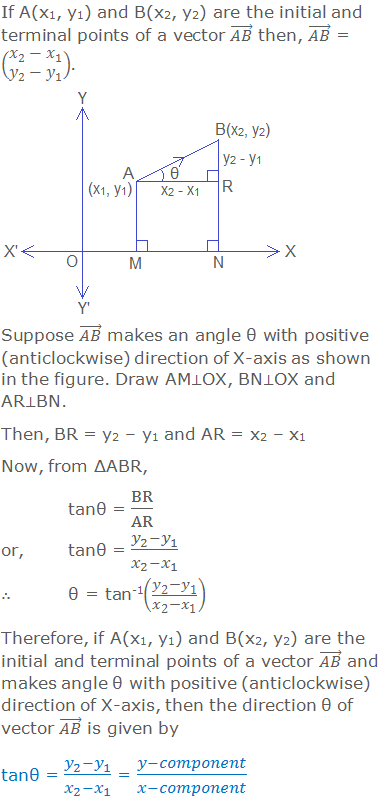# Magnitude and Direction of a Vector## Magnitude of a Vector

The magnitude of a vector is the length of its directed line segment from the initial point to the terminal point. So, the magnitude of a vector is a positive number which is the measure of the line segment representing that vector. The magnitude of a vector is also known as the modulus or absolute value of the vector.

********************

10 Math Problems officially announces the release of Quick Math Solver, an Android App on the Google Play Store for students around the world.

********************## Direction of a Vector

Direction of a vector is the direction of the line segment representing the vector. The direction of a vector is measured as the angle made by the vector with positive (i.e anticlockwise) direction of X-axis.Therefore, for the direction of a vector, we find the tangent of the angle (θ) made by the given vector with the X-axis in a positive (anticlockwise) direction.### Worked Out Examples#### Do you have any questions regarding the magnitude and direction of a vector?

You can ask your questions or problems here, in the comment section below.